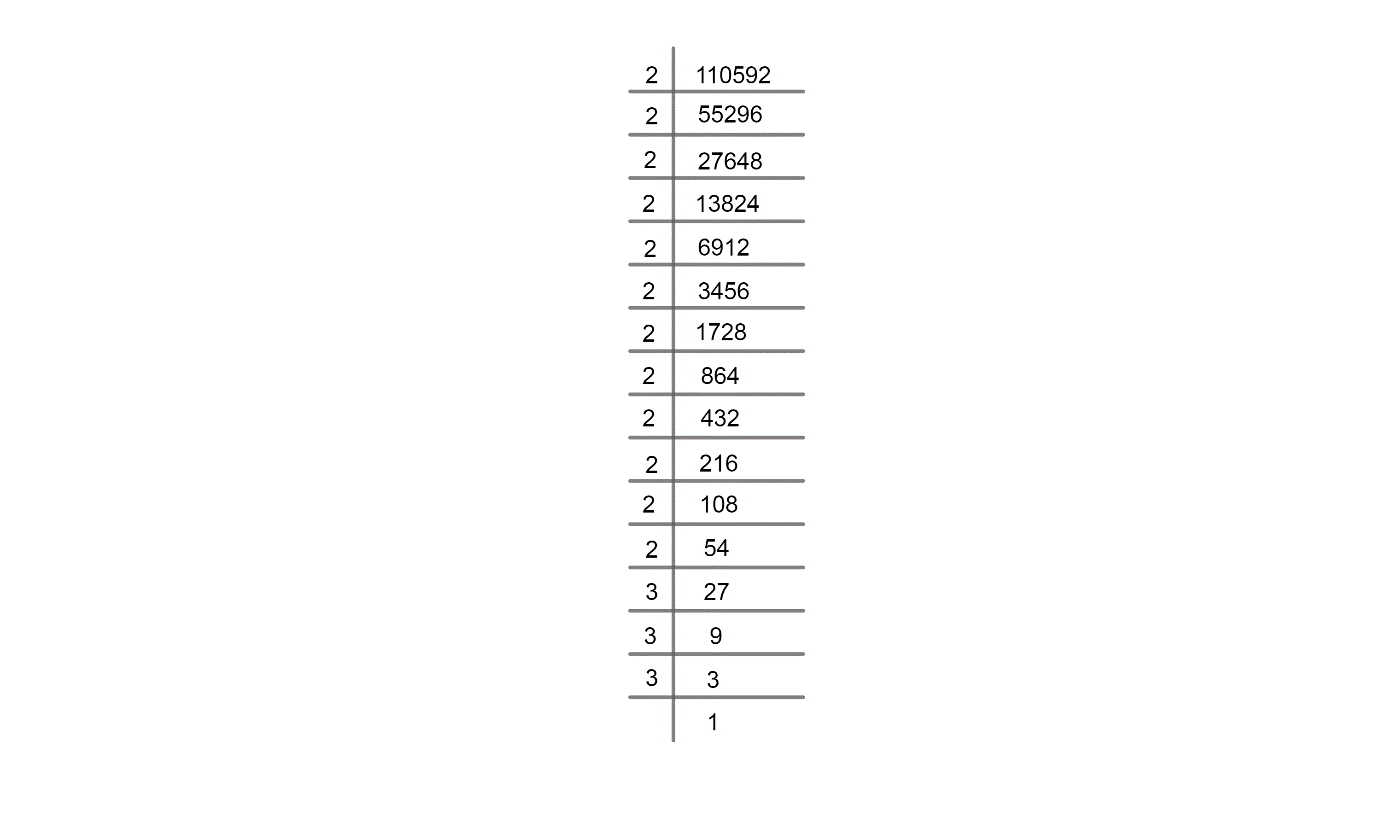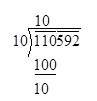QUESTION

# Find cube root of 110592.

Hint: We have to find the cube root of 110592 which means we have to find a number which when multiplied three times produces 110592. Find prime factorization of 110592 and then graph them in blocks of the same factor and take one factor per block and multiply them together to get the cube root.

Given number is 110592.

We have to find the cube root of 110592. It means we have to find a number which will produce 110592, when multiplied three times.

We will use a prime factorization method for finding cube roots.

Step 1: Find prime factorization of given number

$\Rightarrow$ Prime factorization of 110592$\therefore 110592={{2}^{12}}\times {{3}^{3}}$
Step 2: If a number occurs equal to or more than 3 times, since we need to find cube root, we will divide the prime factor in the blocks of three time occurrences i.e.;

\begin{align} & 110592=\underbrace{2\times 2\times 2}_{{}}\times \underbrace{2\times 2\times 2}_{{}}\times \underbrace{2\times 2\times 2}_{{}}\times \underbrace{2\times 2\times 2}_{{}} \\ & \ \ \ \ \ \ \ \ \ \ \ \ \ \ \ \ \ \ \ \ \ \ \ \ \ \ \ \ \ \ \underbrace{3\times 3\times 3}_{{}} \\ \end{align}

And take the number one time in cube root if it is occurring three times in the given number and so on.

Thus, cube root of $110592=2\times 2\times 2\times 2\times 3$

$=48$

Note: Another method that can be used for finding cube root,Short trick for finding cubes;

Step 1: Check the unit digit of the number. It is ‘2’ in this case

$110$

Step 2: The unit digit of cube of ‘8’ ends in 2. Hence, the unit digit of the required cube root will be 8.

${{\left( 1 \right)}^{3}}=1,{{\left( 2 \right)}^{3}}=8,{{\left( 3 \right)}^{3}}=27,{{\left( 4 \right)}^{3}}=64,{{\left( 5 \right)}^{3}}=125,{{\left( 6 \right)}^{3}}=216,{{\left( 7 \right)}^{3}}=343,{{\left( 8 \right)}^{3}}=512,{{\left( 9 \right)}^{3}}=729,{{\left( 10 \right)}^{3}}=1000$We can see that only cube root of ‘8’ ends with ‘2’.

${{8}^{3}}=512$, so the last three digits (i.e. 592) will be covered by ${{8}^{3}}$.

Step 3: Now, look at the remaining digits i.e. the number formed by the first three digits i.e. 110 in this case.

Now, find the largest number whose cube is less than 110.

It will be 4, hence, the tens digit will be 4.

$\left( {{4}^{3}} \right)=64$ which less than 110, and

$\left( {{5}^{3}} \right)=125$which is greater than 110.

So, the required cube root of 110592 is 48.